• Home >
• Probability & Statistics Seminar

The Probability and Statistics seminar is a meeting of the research teams of Prof. Baraud, Prof. Nourdin, Prof. Peccati, Prof. Podolskij and Prof. Thalmaier. Its aim is to present both research works and surveys of mathematical areas of common interest. An archive of talks before 2020 can be seen here.

#### Upcoming sessions

• Thursday 02.12.2021, 1pm, webex

Béatrice Laurent-Bonneau (INSA-Toulouse),  Aggregated tests of independence based on HSIC measures

Abstract: Independence measures based on Reproducing Kernel Hilbert Spaces, also known as Hilbert-Schmidt Independence Criterion and denoted HSIC, are widely used to statistically decide whether or not two random vectors are dependent since the seminal work by [Gretton et al., 2005]. Non-parametric HSIC-based statistical tests of independence have been performed, see [Gretton et al., 2008]. However, these tests lead to the question of the choice of the kernels associated to the HSIC. In particular, there is as yet no method to objectively select specific kernels with theoretical guarantees in terms of first and second kind errors. One of the main contributions of this work is to develop a new HSIC-based aggregated procedure which avoids such a kernel choice, and to provide theoretical guarantees for this procedure. To achieve this, we first introduce non-asymptotic single tests based on Gaussian kernels with a given bandwidth, which are of prescribed level α ∈ (0, 1). From a theoretical point of view, we upper-bound their uniform separation rate of testing over Sobolev and Nikol’skii balls. The key tools to obtain the theoretical performances of the test are exponential inequalities for U-statistics due to [Arcones and Giné, 1993] and [Giné et al., 2000]. Then, we aggregate several single tests, and obtain similar upper-bounds for the uniform separation rate of the aggregated procedure over the same regularity spaces. Another main contribution is that we provide a lower-bound for the non-asymptotic minimax separation rate of testing over Sobolev balls, and deduce that the aggregated procedure is adaptive in the minimax sense over such regularity spaces. The non-asymptotic lower bound is based on the work by [Baraud, 2002]. Finally, from a practical point of view, we perform numerical studies in order to assess the efficiency of our aggregated procedure and compare it to existing independence tests in the literature, in particular to the statistical test of independence based on the kernel mutual information recently studied by [Berrett and Samworth, 2017]. The paper is available on Hal [Albert et al., 2020].

#### Past sessions

• Thursday 18.11.2021, 1pm, webex

Sumit Mukherjee(Columbia University),  Asymptotic distribution of quadratic form

Abstract: In this talk we will give an exact characterization for the asymptotic distribution of quadratic forms in IID random variables with finite second moment, where the underlying matrix is the adjacency matrix of a graph. In particular we will show that the limit distribution of such a quadratic form can always be expressed as the sum of three independent components: a Gaussian, a (possibly) infinite sum of centered chi-squares, and a Gaussian with a random variance. As a consequence, we derive necessary and sufficient conditions for asymptotic normality, and universality of the limiting distribution.

• Thursday 11.11.2021, 1pm, TBA

Denis Belomestny(Duisburg-Essen University)Rates of convergence for density estimation with generative adversarial networks

Abstract: In this work we undertake a thorough study of the non-asymptotic properties of the vanilla generative adversarial networks (GANs). We derive theoretical guarantees for the density estimation with GANs under a proper choice of the deep neural networks classes representing generators and discriminators. In particular, we prove that the resulting estimate converges to the true densityin terms of Jensen-Shannon (JS) divergence at the rate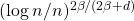where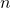is the sample size and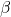determines the smoothness of. To the best of our knowledge, this is the first result in the literature on density estimation using vanilla GANs with JS convergence rates faster than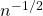in the regime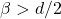. Moreover, we show that the obtained rate is minimax optimal for the considered class of densities.

• Thursday 04.11.2021, 1pm, MSA 3.540

Anatoli Juditsky(Université Grenoble-Alpes), Adaptive estimation from indirect observations

Abstract: We discuss an approach to estimate aggregation and adaptive estimation based upon (nearly optimal) testing of convex hypotheses. We show that in the situation where the observations stem from simple observation schemes (i.e, have Gaussian, discrete and Poisson distribution) and where the set of unknown signals is a finite union of convex and compact sets, the proposed approach leads to aggregation and adaptation routines with nearly optimal performance. As an illustration, we consider application of the proposed estimates to the problem of recovery of unknown signal known to belong to a union of (sic) in Gaussian observation scheme. The corresponding numerical routines can be implemented efficiently when the number of sets in the union is “not very large”. We illustrate the “practical performance” of the method in a numerical example of estimation in the single index model.

• Thursday 14.10.2021, 1pm, MSA auditorium 3.530

Elisabeth Gassiat (Université Paris-Saclay), Deconvolution with unknown noise distribution

Abstract: I consider the deconvolution problem in the case where no information is known about the noise distribution. More precisely, no assumption is made on the noise distribution and no samples are available to estimate it: the deconvolution problem is solved based only on observations of the corrupted signal. I will prove the identifiability of the model up to translation when the signal has a Laplace transform with an exponential growth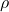smaller than 2 and when it can be decomposed into two dependent components, so that the identifiability theorem can be used for sequences of dependent data or for sequences of iid multidimensional data.  In the case of iid multidimensional  data, I will propose an adaptive estimator of the density of the signal and provide rates of convergence. This rate of convergence is known to be minimax when ρ = 1.

• Thursday 01.07.2021, 1pm, Webex

Christoph Thäle (Ruhr Universität Bochum)Poisson hyperplanes in hyperbolic space

Abstract: In the focus of this talk are random tessellations in hyperbolic space induced by Poisson point processes on the space of hyperbolic hyperplanes (totally geodesic subspaces of co-dimension 1). In the first part of the talk we consider the so-called k-skeleton of such tessellations and prove that, when observed in a sequence of increasing observation windows, the k-volume of the k-skeleton satisfies a central limit theorem only for dimensions 2 and 3 and that asymptotic normality fails in all higher dimensions. We indicate possible generalizations to Poisson processes of lower-dimensional random subspaces as well. If time permits we also describe a way to address the combinatorial structure of the zero cell of a hyperbolic hyperplane tessellation. In particular we present a fully explicit formula for the number of facets of this cell.

• Thursday 24.06.2021, 1pm, Webex

Maurizia Rossi (University of Milano-Bicocca)Non-universal fluctuations of the empirical measure for sphere-cross-time random fields

Abstract: In this talk we consider isotropic and stationary real Gaussian sphere-cross-time random fields and we investigate the large time asymptotic behavior of the empirical measure at any threshold, covering both cases when the field exhibits short and long memory, i.e. integrable and non-integrable temporal covariance. It turns out that the limiting distribution is not universal, depending both on the memory parameters and the threshold. In particular, in the long memory case a form of Berry’s cancellation phenomenon occurs at zero-level, inducing phase transitions for both variance rates and limiting laws. (This talk is based on a joint work with D. Marinucci and A. Vidotto.)

• Thursday 10.06.2021, 1pm, Webex

Yvik Swan (Université libre de Bruxelles) Stein’s density method for multivariate continuous distributions

Abstract: We will discuss a general framework for Stein’s density method for multivariate continuous distributions. The approach associates to any probability density function a canonical operator and Stein class, as well as an infinite collection of operators and classes which we call standardizations. These in turn spawn an entire family of Stein identities and characterizations for any continuous distribution on Rd, among which we highlight those based on the score function and the Stein kernel. A feature of these operators is that they do not depend on normalizing constants. A new definition of Stein kernel is introduced and examined; integral formulas are obtained through a connection with mass transport, as well as ready-to-use explicit formulas for elliptical distributions. The flexibility of the kernels is used to compare in Stein discrepancy (and therefore 2-Wasserstein distance) between two normal distributions, Student and normal distributions, as well as two normal-gamma distributions. Upper and lower bounds on the 1-Wasserstein distance between continuous distributions are provided, and computed for a variety of examples: comparison between different normal distri- butions (improving on existing bounds in some regimes), posterior distributions with different priors in a Bayesian setting (including logistic regression), centred Azzalini- Dalla Valle distributions. Finally the notion of weak Stein equation and weak Stein factors is introduced, and new bounds are obtained for Lipschitz test functions if the distribution admits a Poincaré constant, which we use to compare in 1-Wasserstein distance between different copulas on the unit square. (arXiv : https://arxiv.org/abs/2101.05079)

• Thursday 27.05.2021, 1pm, Webex

Louis Gass (IRMAR)A Salem Zygmund approach to almost sure asymptotics concerning the nodal measure of Riemannian random waves

Abstract: Let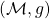be a compact, boundaryless Riemannian manifold, and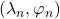the sequences of (ordered) Laplace eigenvalues and eigenfunctions, satysfying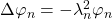. We consider the model of Riemannian random waves defined by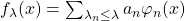, where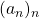is a iid sequence of Gaussian random variables. With probability one with respect to the Gaussian coefficients, we establish that the process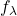, properly rescaled and evaluated at an independently and uniformly chosen point X on the manifold, converges in distribution towards an universal Gaussian field as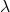grows to infinity. Using the continuity of the nodal measure with respect to the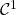topology, we deduce that almost surely with respect to the Gaussian coefficient, the nodal measure of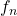weakly converges towards the Riemannian volume.

• Thursday 20.05.2021, 1pm, Webex

Anna Gusakova (Ruhr-Universität Bochum)Random simplicial tessellations: high-dimensional probabilistic behaviour of the typical cells

Abstract: A tessellation in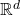is a locally finite collection of convex polytopes, which cover the space and have disjoin interior. In this talk we consider a few models of random simplicial tessellations, the so-called Delaunay tessellations, whose construction is based on Poisson point process. Among them is the classical Poisson-Delaunay tessellation.
The main object we are interested in is the typical cell of a random tessellation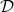. Intuitively, one can think of it as a randomly chosen polytope from the collection, assuming that each polytope has the same chance to be chosen. Considering the volume of the typical cells of our models we derive the explicit formulas for the moments as well as probabilistic representation in term of independent gamma- and beta-distributed random variables. Moreover, we investigate the limiting probabilistic behaviour of the logarithmic volume of typical cell, when dimension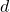tends to infinity. In particular we establish central limit theorem and large deviation principle.

• Thursday 06.05.2021, 1pm, Webex

Hugo Vanneuville (Université Grenoble-Alpes)The percolation phase transition of the random plane wave

Abstract: Consider the random plane wave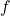, which is a random eigenfunction of the Laplacian in. Given a real number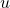, we study the connectivity properties of the set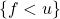, and we show that the model undergoes a percolation phase transition at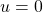: if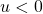then a.s. there is no unbounded connected component inwhile this is a.s. the case if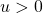. As I will explain in the talk, the main difficulty is that the field is not positively correlated. In the talk, I will present the strategy of proof, based on some superconcentration considerations that have enabled us to revisit the following general idea from (Russo, 1982; Talagrand, 1994…): “an event satisfies a phase transition if it depends little on any given point”. This is joint work with Stephen Muirhead and Alejandro Rivera.

• Thursday 22.04.2021, 1pm, Webex

Vytauté Pilipauskaite (University of Luxembourg)Local scaling limits of Lévy driven fractional random fields

Abstract: We obtain all local scaling limits for a class of Lévy driven fractional random fields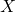on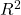. More specifically, the random fieldis defined as integral of a non-random function with respect to infinitely divisible random measure. The scaling procedure involves increments of X over points between which the distance in the horizontal and vertical directions shrinks respectively as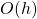and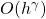as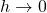for a given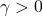. We consider two types of increments of: usual increment and rectangular increment. We call their local scaling limits respectively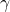-tangent and-rectangent random fields. We show that for aboveboth types of local scaling limits exist for anyand undergo a transition at some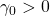. We also discuss properties of these limits. This is a joint work with Donatas Surgailis (Vilnius University, Lithuania).

• Thursday 18.03.2021, 1pm, Webex

Etienne Roquain (LPSM)False Discovery Rate control with unknown null distribution

Abstract: Classical multiple testing theory prescribes the null distribution, which is often a too stringent assumption for nowadays large scale experiments. This paper presents theoretical foundations to understand the limitations caused by ignoring the null distribution, and how it can be properly learned from the (same) data-set, when possible. While an oracle procedure in that case is the Benjamini Hochberg procedure applied with the true (unknown) null distribution, we pursue the aim of building a procedure that asymptotically mimics the performance of the oracle (AMO in short). For a Gaussian null, our main result states that an AMO procedure exists if and only if the sparsity parameter k (number of false nulls) is of order less than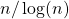, where n is the total number of tests.
This is a joint work with Nicolas Verzelen, https://arxiv.org/abs/1912.03109.

• Thursday 11.03.2021, 1pm, Webex

Chiara Amorino (University of Luxembourg)Rate of estimation for the stationary distribution of jump-processes over anisotropic Holder classes

Abstract: We consider the solution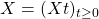of a multivariate stochastic differential equation with Levy-type jumps and with unique invariant probability measure
with density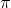. We assume that a continuous record of observations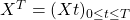is available.
In the case without jumps, under respectively isotropic and anisotropic Holder smoothness constraints, Dalalyan and Reiss  and Strauch  have found convergence rates of invariant density estimators which are considerably faster than those known from standard multivariate density estimation.
We extend the previous works by obtaining, in presence of jumps and in an anisotropic context, some estimators which achieve the continuous convergence rates in mono and bi-dimensional cases and a convergence rate faster than the ones found by Strauch  for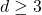.
Moreover, we obtain a minimax lower bound on the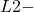risk for pointwise estimation, with the same rate up to a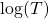term. It implies that, on a class of diffusions whose invariant density belongs to the anisotropic Holder class we are considering, it is impossible to find an estimator with a rate of estimation faster than the one we propose.
References
 Dalalyan, A. and Reiss, M. (2007). Asymptotic statistical equivalence for ergodic diffusions: the multidimensional case. Probab. Theory Relat. Fields, 137(1), 25-47.
 Strauch, C. (2018). Adaptive invariant density estimation for ergodic diffusions over anisotropic classes. The Annals of Statistics, 46(6B), 3451-3480. 1

• Thursday 25.02.2021, 2pm, TBA

Richard Samworth (University of Cambridge)USP: an independence test that improves on Pearson’s chi-squared and the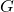-test

Abstract: We introduce the U-Statistic Permutation (USP) test of independence in the context of discrete data displayed in a contingency table. Either Pearson’s chi-squared test of independence, or the Generalised Likelihood Ratio test (G-test), are typically used for this task, but we argue that these tests have serious deficiencies, both in terms of their inability to control the size of the test, and their power properties. By contrast, the USP test is guaranteed to control the size of the test at the nominal level for all sample sizes, has no issues with small (or zero) cell counts, and is able to detect distributions that violate independence in only a minimal way. The test statistic is derived from a U-statistic estimator of a natural population measure of dependence, and we prove that this is the unique minimum variance unbiased estimator of this population quantity.
In the last one-third of the talk, I will show how this is a special case of a much more general methodology and theory for independence testing.